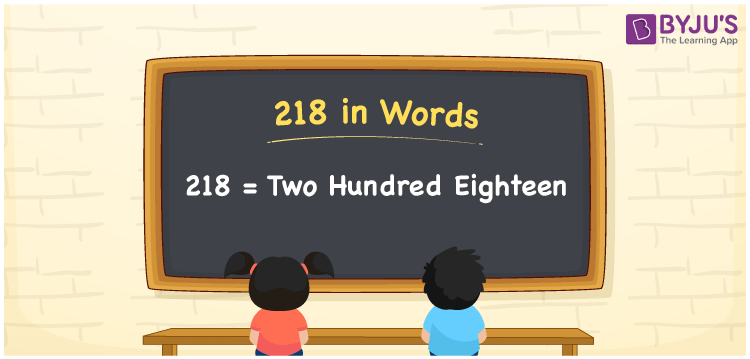# 218 in Words

218 in words is written as Two Hundred Eighteen. The name of number 218 in English is “Two Hundred Eighteen”. The word Two Hundred Eighteen is used in many real-life situations. For example, there are Two Hundred Eighteen students in a classroom. Thus, it is a counting number. Also, to represent the currency equal to 218, we can write it in words as Rupees Two Hundred Eighteen or Two Hundred Eighteen rupees. Therefore, it is necessary to learn the numbers in words, for the ease of understanding and expressing them.

 218 in words Two Hundred Eighteen Two Hundred Eighteen in Numbers 218

## 218 in English Words## How to Write 218 in Words?

If we know the place value of digits of 218, then we can easily express it in words. The place value is basically the position of a digit in a number. 218 is a three-digit number, therefore, we can specify the position of each digit of 218 in a place value chart. In the Indian numbering system, the order of place value of digits from right to left is given by:

 Hundreds Tens Ones 2 1 8

We can write it in expanded form as:

2 × Hundred + 1 × Ten + 8 × One

= 2 × 100 + 1 × 10 + 8 × 1

= 200 + 10 + 8

= 218

= Two Hundred Eighteen

Therefore, 218 in words is written as Two Hundred Eighteens.

218 is a natural number that precedes 219 and succeeds 217.

218 in words – Two Hundred Eighteen

Is 218 an odd number? – No

Is 218 an even number? – Yes

Is 218 a perfect square number? – No

Is 218 a perfect cube number? – No

Is 218 a prime number? – No

Is 218 a composite number? – Yes

## Frequently Asked Questions on 218 in Words

Q1

### How to write 218 in words?

218 in English is written as “Two Hundred Eighteen”.
Q2

### Is 218 an odd number or even number?

218 is an even number because it is wholly divisible by 2.
218/2 = 109
Q3

### Write Two Hundred Eighteen in numbers.

Two Hundred Eighteen in numbers is 218.